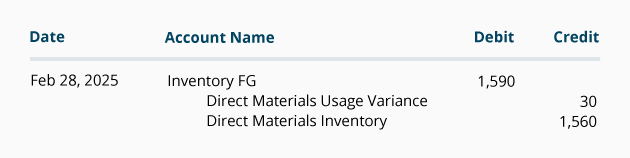# Direct Materials Usage Variance

In a standard costing system, the costs of production, inventories, and the cost of goods sold are initially recorded using the standard costs. In the case of direct materials, it means the standard quantity of direct materials that should have been used to make the good output. If the manufacturer uses more direct materials than the standard quantity of materials for the products actually manufactured, the company will have an unfavorable direct materials usage variance. If the quantity of direct materials actually used is less than the standard quantity for the products produced, the company will have a favorable usage variance. The amount of a favorable and unfavorable variance is recorded in a general ledger account Direct Materials Usage Variance. (Alternative account titles include Direct Materials Quantity Variance or Direct Materials Efficiency Variance.) We will demonstrate this variance with the following information.

### January 2022

In order to calculate the direct materials usage (or quantity) variance, we start with the number of acceptable units of products that have been manufactured—also known as the good output. At DenimWorks this is the number of good aprons physically produced. If DenimWorks produces 100 large aprons and 60 small aprons during January, the production and the finished goods inventory will begin with the cost of the direct materials that should have been used to make those aprons. Any difference will be a variance.

Note:

We are not determining the quantity of aprons that DenimWorks should have made. Rather, we are determining whether the 100 large aprons and 60 small aprons that were actually manufactured were produced efficiently. In the case of direct materials, we want to determine whether or not the company used the proper amount of denim to make the 160 aprons that were actually produced. (For the purposes of calculating the direct materials usage variance, it doesn't matter whether DenimWorks had a goal to produce 100 aprons, 200 aprons, or 250 aprons. The direct materials usage variance is computed for the actual number of aprons produced.)

Standard costs are sometimes referred to as the "should be costs." DenimWorks should be using 278 yards of denim to make 100 large aprons and 60 small aprons as shown in the following table.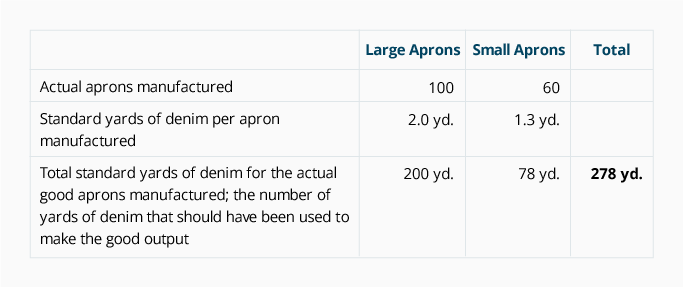We determine the total standard cost of the denim that should have been used to make the 160 aprons by multiplying the standard quantity of denim (278 yards) by the standard cost of a yard of denim (\$3 per yard):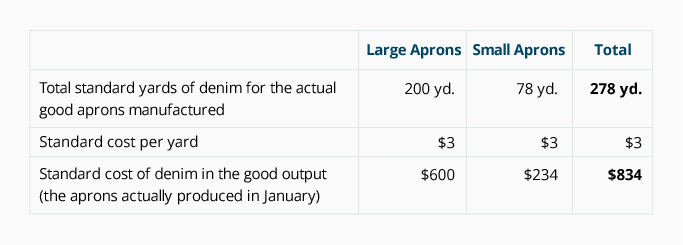An inventory account (such as F.G. Inventory or Work-in-Process) is debited for \$834; this is the standard cost of the direct materials component in the aprons manufactured in January 2022.

The Direct Materials Inventory account is reduced by the standard cost of the denim that was removed from the direct materials inventory. Let's assume that the actual quantity of denim removed from the direct materials inventory and used to make the aprons in January was 290 yards. Because Direct Materials Inventory reports the standard cost of the actual materials on hand, we reduce the account balance by \$870 (290 yards used \$3 standard cost per yard). After removing 290 yards of materials, the balance in the Direct Materials Inventory account as of January 31 is \$2,130 (710 yards x \$3 standard cost per yard).

The Direct Materials Usage Variance is: [the standard quantity of material that should have been used to make the good output minus the actual quantity of material used] X the standard cost per yard.

In our example, DenimWorks should have used 278 yards of material to make 100 large aprons and 60 small aprons. Because the company actually used 290 yards of denim, we say that DenimWorks did not operate efficiently. When we multiply the additional 12 yards times the standard cost of \$3 per yard, the result is an unfavorable direct materials usage variance of \$36.

For the remainder of our explanation, we will use a common format for calculating variances. The amounts for each column are computed in the order indicated in the headings.

Direct Materials Usage/Quantity/Efficiency Variance Analysis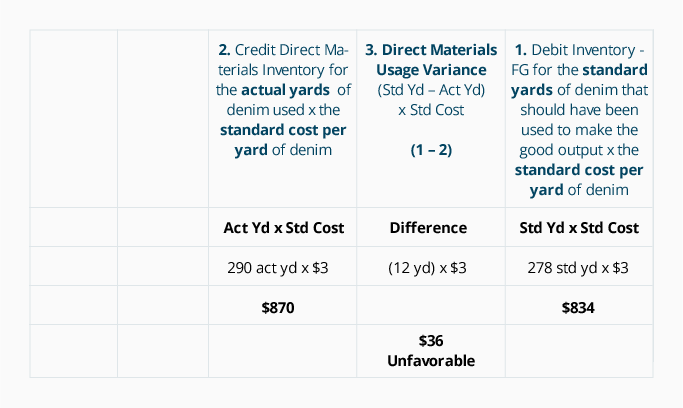The journal entry for the direct materials used for the January production is: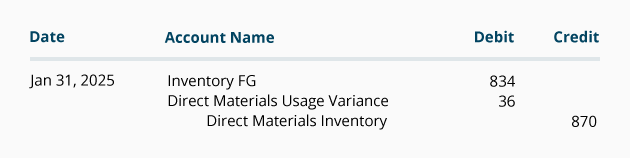### February 2022

Let's assume that in February 2022 DenimWorks produces 200 large aprons and 100 small aprons and that 520 yards of denim are actually used. From this information we can compute the following: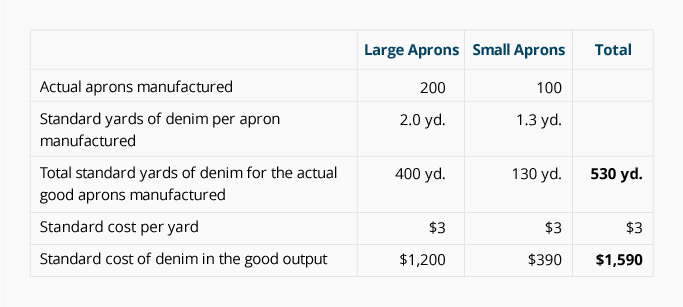Let's put the February 2022 information into our format:

Direct Materials Usage (or Quantity) Variance Analysis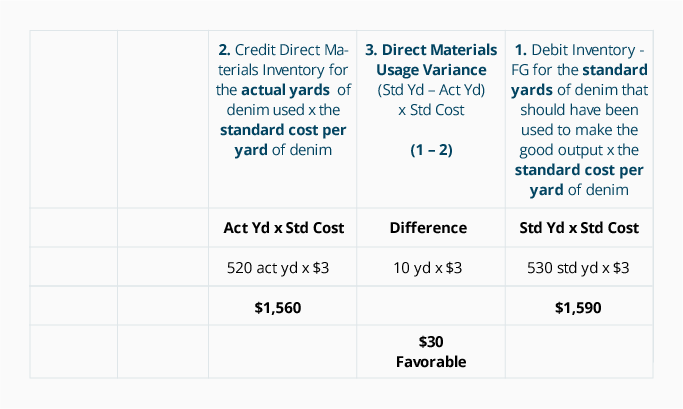The journal entry for the direct materials used for the February production is: